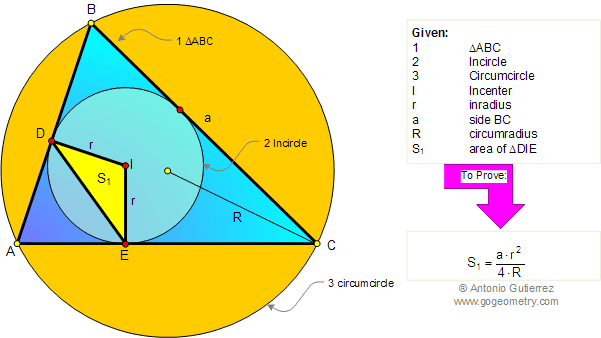# Geometry Problem 81: Triangle Area, Side, Inradius, Circumradius. High School, College, Math Education

 Given a triangle ABC, the incircle of center I, inradius r, and circumradius R. If BC = a, prove that area of triangle DIE is:  $$S_1 = \dfrac{a\cdot r^2}{4\cdot R}$$.Home | Search | Geometry | Problems | All Problems | Open Problems | Visual Index | 81-90 | Circle Tangent Line | Email | View or post a solution | by Antonio Gutierrez